# Test: Limits And Continuity : Intuitive Approach - 1

## 40 Questions MCQ Test Quantitative Aptitude for CA CPT | Test: Limits And Continuity : Intuitive Approach - 1

Description
Attempt Test: Limits And Continuity : Intuitive Approach - 1 | 40 questions in 40 minutes | Mock test for CA Foundation preparation | Free important questions MCQ to study Quantitative Aptitude for CA CPT for CA Foundation Exam | Download free PDF with solutions
QUESTION: 1

Solution:
QUESTION: 2

Solution:
QUESTION: 3

### If g(x) = 3 – x2 then g(x) is

Solution:
QUESTION: 4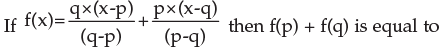Solution:
QUESTION: 5

If f(x) = 2x2 – 5x + 4 then 2f(x) = f(2x) for

Solution:
QUESTION: 6

If f(x) = logx (x > 0) then f(p) + f(q) +f(r) is

Solution:
QUESTION: 7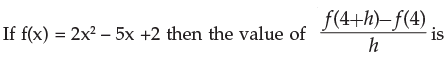Solution:
QUESTION: 8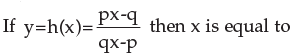Solution:
QUESTION: 9

If f(x) = x2 – x then f( h+1) is equal to

Solution:
QUESTION: 10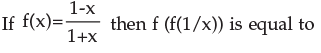Solution:
QUESTION: 11

f (x) is a continuous function and takes only rational values. If f (0) = 3, then f (2) equals

Solution:
QUESTION: 12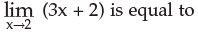Solution:
QUESTION: 13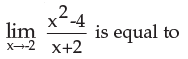Solution:
QUESTION: 14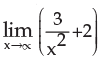Solution:
QUESTION: 15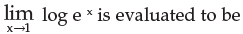Solution:
QUESTION: 16

The value of the limit of f(x) as x →3 when f(x)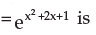Solution:
QUESTION: 17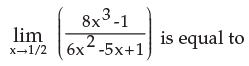Solution:
QUESTION: 18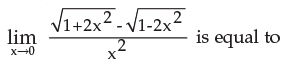Solution:
QUESTION: 19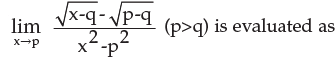Solution:
QUESTION: 20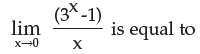Solution:
QUESTION: 21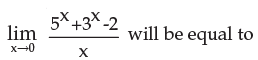Solution:
QUESTION: 22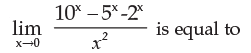Solution:
QUESTION: 23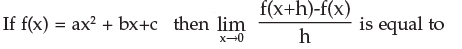Solution:
QUESTION: 24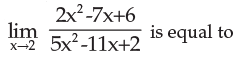Solution:
QUESTION: 25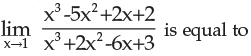Solution:
QUESTION: 26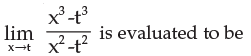Solution:
QUESTION: 27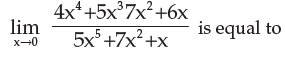Solution:
QUESTION: 28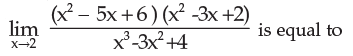Solution:
QUESTION: 29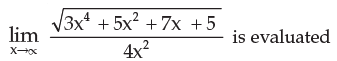Solution:
QUESTION: 30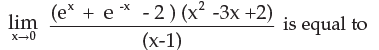Solution:
QUESTION: 31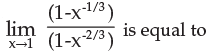Solution:
QUESTION: 32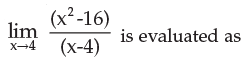Solution:
QUESTION: 33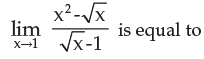Solution:
QUESTION: 34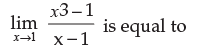Solution:
QUESTION: 35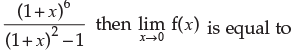Solution:
QUESTION: 36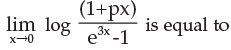Solution:
QUESTION: 37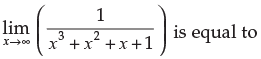Solution:
QUESTION: 38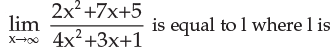Solution:
QUESTION: 39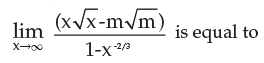Solution:
QUESTION: 40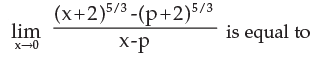Solution:Use Code STAYHOME200 and get INR 200 additional OFF Use Coupon Code